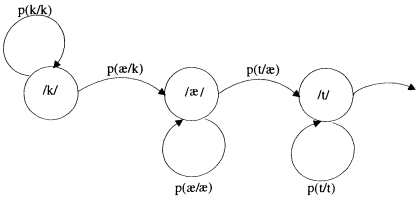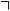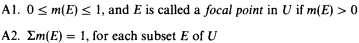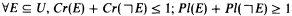Rating : ⭐⭐⭐⭐⭐
Price : \$10.99
Language:EN
Pages: 2

# Basic probability assignment bpa

Page 140Probabilities have some limitations. One is that they cannot represent ignorance. An event has to be either "present" or "not present" at every moment. If we don't know anything about the event we will have difficulty using probabilities. Some theories have extended the probability theory to overcome this limitation. Such theories are, for example, the Dempster-Shafer theory, certainty factors, and possibility theory. Some of them do not provide operational definitions for the new probability terms, that is, the definition does not necessarily state the way to find out the values for the parameters of uncertainty. But some axioms are given with formulas for calculating how to operate with a set of data or rules with parameters of uncertainty assigned to them.The main idea is to represent the uncertainties of an event E not as a single probability p(E), but as an interval of lower probability and a higher probability. The following axioms are held for the Dempster-Shafer theory:It has been proved as a corollary thatHow It Works